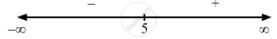PUC Karnataka Science Class 12Department of Pre-University Education, Karnataka
Share

F(X) = (X − 5)4. - PUC Karnataka Science Class 12 - Mathematics

Question

f(x) = (x $-$ 5)4.

Solution

$\text { Given: } \hspace{0.167em} f\left( x \right) = \left( x - 5 \right)^4$

$\Rightarrow f'\left( x \right) = 4 \left( x - 5 \right)^3$

$\text { For a local maximum or a local minimum, we must have }$

$f'\left( x \right) = 0$

$\Rightarrow 4 \left( x - 5 \right)^3 = 0$

$\Rightarrow x = 5$Since f '(x) changes from negative to positive when x increases through 5, x = 5 is the point of local minima.
The local minimum value of  f (x) at x = 5 is given by $\left( 5 - 5 \right)^4 = 0$ .

Is there an error in this question or solution?

Video TutorialsVIEW ALL 

Solution F(X) = (X − 5)4. Concept: Graph of Maxima and Minima.
S